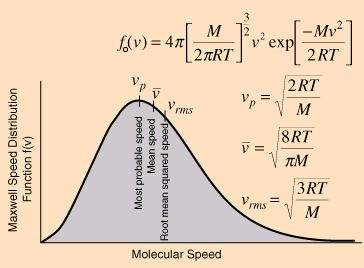# Maxwell and The distribution of velocities in a gas

• I
Aleoa
In the first volume of his lectures (cap 6-4) Feynman presents Maxwell's distribution of velocities of the molecules in a gas.And, referring to the PDF graph he says:

"If we consider the molecules in a typical container (with a volume of, say, one
liter), then there are a very large number N of molecules present (N ≈ $10^{22}$).
Since p(v) ∆v is the probability that one molecule will have its velocity in ∆v,
by our definition of probability we mean that the expected number <∆N> to be
found with a velocity in the interval ∆v is given by: $<\Delta N>=Np(v)\Delta v$.
[...]
Since with a gas we are usually dealing with large numbers of molecules,
we expect the deviations from the expected numbers to be small (like 1/√N), so
we often neglect to say the “expected” number
"

I have not understand this part, how it's possible to derive this 1/√N deviation ( or a value the gives the idea of what Feynman is saying) ?

#### Attachments

willem2
I have not understand this part, how it's possible to derive this 1/√N deviation ( or a value the gives the idea of what Feynman is saying) ?
This is the central limit theorem. If you have N identically distributed random variables with mean μ and variance σ2, the average of the variables will approach a normal distribution for large N with mean μ and variance σ2/N. The standard deviation will be $\frac {\sigma}{\sqrt N}$. The average speed of the molecules is such a set of random variables, but you can also use a variable that is 1 if the speed is in a certain range, and 0 if it is not, and you can use this to calculate the standard deviation for the number of molecules in a certain velocity range,

•Aleoa
Aleoa
This is the central limit theorem. If you have N identically distributed random variables with mean μ and variance σ2, the average of the variables will approach a normal distribution for large N with mean μ and variance σ2/N. The standard deviation will be $\frac {\sigma}{\sqrt N}$. The average speed of the molecules is such a set of random variables, but you can also use a variable that is 1 if the speed is in a certain range, and 0 if it is not, and you can use this to calculate the standard deviation for the number of molecules in a certain velocity range,

I'm not able to understand which is , in this case, the average random variable that is subjected to CLT ...
How does the bold phrase in the main post concern to the distribution of sample mean ?

Last edited:
Homework Helper
I think there's a step missed out (assumed), and a slight error. If the probability of a single molecule having a velocity in the range v → v + Δv is p(v)Δv, then for N molecules, the number with a velocity in that range follows a binomial distribution, with a mean (expectation value) of Np(v)Δv and a variance of Np(v)Δv(1-p(v)Δv), which is approximately Np(v)Δv if p(v)Δv << 1. So the standard deviation is √(Np(v)Δv); note that this increases as √N (the error in the statement above). The fraction of molecules with velocities in the range Δv has an expectation value Np(v)Δv/N = p(v)Δv, and a standard deviation of √(p(v)Δv/N). This is the "deviation that goes like 1/√N".

I'm not able to understand which is , in this case, the average random variable that is subjected to CLT ...

Assume each of ##N## particles has a independent probability of ##p(v_1) dv## of having (at a given time) its velocity in ##I_1## and probability of ##1 - p(v_1)dv## of having its velocity in some other interval. Whether a particle has a velocity in ##I## is a Bernoulli random variable with probability of success equal to ##p(v_1)dv##.

The number of particles (at a given time) that have their velocities in ##I_1## is a binomial random variable ##B_1## . The random variable ##B_1## is the sum of ##N## identical independent Bernoulli random variables.

Feynman's remark says that (at a given time) the number of particles that have their velocities in ##I_1## has a high probability of being close to the mean of ##B_1##, which is ##N p(v_1) dv##.

There are some technicalities to consider.

1. Does "close" mean close in the absolute sense - e.g. within plus or minus 2? Or does "close" mean in the sense of a percentage - e.g. within plus or minus 2% of ##N##?

2. The analysis for one interval ##I_1## is a not joint result about what happens for two different intervals ##I_1,I_2##. Whether a particle has a velocity in ##I_1## is not independent of whether it has a velocity in ##I_2##.
Feynman's claim is that (jointly) for each interval ##I_j## the number of particles with velocities in that interval has a high probability of being ##Np(v_j) dv##.

Of course, physicists don't always worry about mathematical technicalities!

Last edited: